##### Question

In: Statistics and Probability

# Let x be a random variable that represents the level of glucose in the blood (milligrams...

Let x be a random variable that represents the level of glucose in the blood (milligrams per deciliter of blood) after a 12 hour fast. Assume that for people under 50 years old, x has a distribution that is approximately normal, with mean μ = 52 and estimated standard deviation σ = 10. A test result x < 40 is an indication of severe excess insulin, and medication is usually prescribed.

(a) What is the probability that, on a single test, x < 40? (Round your answer to four decimal places.)

(b) Suppose a doctor uses the average x for two tests taken about a week apart. What can we say about the probability distribution of x? Hint: See Theorem 6.1.

The probability distribution of x is approximately normal with μx = 52 and σx = 10.The probability distribution of x is approximately normal with μx = 52 and σx = 5.00.     The probability distribution of x is approximately normal with μx = 52 and σx = 7.07.The probability distribution of x is not normal.

What is the probability that x < 40? (Round your answer to four decimal places.)

(c) Repeat part (b) for n = 3 tests taken a week apart. (Round your answer to four decimal places.)

(d) Repeat part (b) for n = 5 tests taken a week apart. (Round your answer to four decimal places.)

(e) Compare your answers to parts (a), (b), (c), and (d). Did the probabilities decrease as n increased?

YesNo

Explain what this might imply if you were a doctor or a nurse.

The more tests a patient completes, the weaker is the evidence for excess insulin.The more tests a patient completes, the weaker is the evidence for lack of insulin.     The more tests a patient completes, the stronger is the evidence for lack of insulin.The more tests a patient completes, the stronger is the evidence for excess insulin.

## Solutions

##### Expert Solution

Solution :

Given that ,

mean =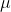= 52

standard deviation =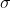= 10

a) P(x < 40) = P[(x -) /< (40 - 52) / 10]

= P(z < -1.20)

Using z table,

= 0.1151

b) n = 2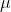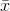== 52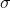=/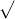n = 10/2 = 7.07

The probability distribution of x is approximately normal with μx = 52 and σx = 7.07

P(< 40) = P((-) /< (40 - 52) / 7.07)

= P(z < -1.70)

Using z table

= 0.0446

c) n = 3== 52=/n = 10/3 = 5.77

The probability distribution of x is approximately normal with μx = 52 and σx = 5.77.

P(< 40) = P((-) /< (40 - 52) / 5.77)

= P(z < -2.08)

Using z table

= 0.0188

d) n = 5== 52=/n = 10/5 = 4.47

The probability distribution of x is approximately normal with μx = 52 and σx = 4.47

P(< 40) = P((-) /< (40 - 52) / 4.47)

= P(z < -2.68)

Using z table

= 0.0037

e) yes,

The more tests a patient completes, the weaker is the evidence for excess insulin

## Related Solutions

##### Let x be a random variable that represents the level of glucose in the blood (milligrams...
Let x be a random variable that represents the level of glucose in the blood (milligrams per deciliter of blood) after a 12-hour fast. Assume that for people under 50 years old, x has a distribution that is approximately normal, with mean μ = 88 and estimated standard deviation σ = 28. A test result x < 40 is an indication of severe excess insulin, and medication is usually prescribed. (a) What is the probability that, on a single test,...
##### Let x be a random variable that represents the level of glucose in the blood (milligrams...
Let x be a random variable that represents the level of glucose in the blood (milligrams per deciliter of blood) after a 12 hour fast. Assume that for people under 50 years old, x has a distribution that is approximately normal, with mean μ = 96 and estimated standard deviation σ = 47. A test result x < 40 is an indication of severe excess insulin, and medication is usually prescribed. (a) What is the probability that, on a single...
##### Let x be a random variable that represents the level of glucose in the blood (milligrams...
Let x be a random variable that represents the level of glucose in the blood (milligrams per deciliter of blood) after a 12 hour fast. Assume that for people under 50 years old, x has a distribution that is approximately normal, with mean μ = 84 and estimated standard deviation σ = 29. A test result x < 40 is an indication of severe excess insulin, and medication is usually prescribed. (a) What is the probability that, on a single...
##### Let x be a random variable that represents the level of glucose in the blood (milligrams...
Let x be a random variable that represents the level of glucose in the blood (milligrams per deciliter of blood) after a 12 hour fast. Assume that for people under 50 years old, x has a distribution that is approximately normal, with mean μ = 60and estimated standard deviation σ = 32. A test result x < 40 is an indication of severe excess insulin, and medication is usually prescribed. (a) What is the probability that, on a single test,...
##### Let x be a random variable that represents the level of glucose in the blood (milligrams...
Let x be a random variable that represents the level of glucose in the blood (milligrams per deciliter of blood) after a 12 hour fast. Assume that for people under 50 years old, x has a distribution that is approximately normal, with mean μ = 86 and estimated standard deviation σ = 35. A test result x < 40 is an indication of severe excess insulin, and medication is usually prescribed. (d) Repeat part (b) for n = 5 tests...
##### Let x be a random variable that represents the level of glucose in the blood (milligrams...
Let x be a random variable that represents the level of glucose in the blood (milligrams per deciliter of blood) after a 12-hour fast. Assume that for people under 50 years old, x has a distribution that is approximately normal, with mean μ = 71 and estimated standard deviation σ = 30. A test result x < 40 is an indication of severe excess insulin, and medication is usually prescribed. (a) What is the probability that, on a single test,...
##### Let x be a random variable that represents the level of glucose in the blood (milligrams...
Let x be a random variable that represents the level of glucose in the blood (milligrams per deciliter of blood) after a 12 hour fast. Assume that for people under 50 years old, x has a distribution that is approximately normal, with mean μ = 56 and estimated standard deviation σ = 42. A test result x < 40 is an indication of severe excess insulin, and medication is usually prescribed. A.) What is the probability that, on a single...
##### Let x be a random variable that represents the level of glucose in the blood (milligrams...
Let x be a random variable that represents the level of glucose in the blood (milligrams per deciliter of blood) after a 12 hour fast. Assume that for people under 50 years old, x has a distribution that is approximately normal, with mean μ = 51 and estimated standard deviation σ = 47. A test result x < 40 is an indication of severe excess insulin, and medication is usually prescribed. (a) What is the probability that, on a single...
##### Let x be a random variable that represents the level of glucose in the blood (milligrams...
Let x be a random variable that represents the level of glucose in the blood (milligrams per deciliter of blood) after a 12 hour fast. Assume that for people under 50 years old, x has a distribution that is approximately normal, with mean μ = 56 and estimated standard deviation σ = 24. A test result x < 40 is an indication of severe excess insulin, and medication is usually prescribed. (a) What is the probability that, on a single...
##### Let x be a random variable that represents the level of glucose in the blood (milligrams...
Let x be a random variable that represents the level of glucose in the blood (milligrams per deciliter of blood) after a 12 hour fast. Assume that for people under 50 years old, x has a distribution that is approximately normal, with mean μ = 51 and estimated standard deviation σ = 47. A test result x < 40 is an indication of severe excess insulin, and medication is usually prescribed. (a) What is the probability that, on a single...